# The problem is to solve optimal control problem by economy optimality for the process

### Содержание работы

3. The problem is to solve optimal control problem by economy optimality for the process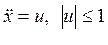for minimum of expences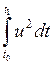when the initial conditions are: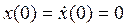,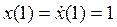.

Solution

1)  First of all we must write phase coordinates: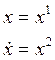So, this process can be described by the system of differential equations: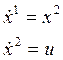where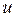- control parameter.

2)  Write Hamilton-Pontryagin function for our case: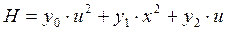,

where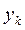- auxiliary functions.

For finding of control parameterlet’s use the maximal principal, which states that in optimal process Hamilton-Pontryagin function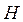gets maximum value, for existing of which the requirement is following: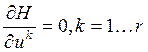So, we have for our case: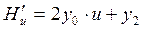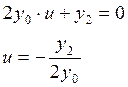For finding of auxiliary functionslet’s use Hamilton-Pontryagin equation in general form are the following: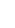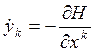Let’s write it for our case: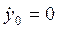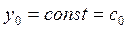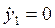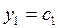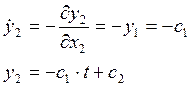Substituting the got values of auxiliary functionsin formula for finding of control parameterwe obtain: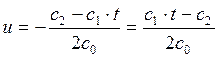,

Let’s denote our constant in more compact view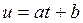where,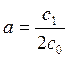,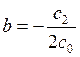.

3)  Let’s return to initial coordinates and synthesize the phase trajectory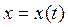.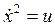, so we have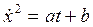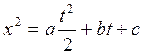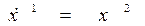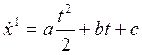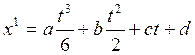4)  Let’s use the initial conditions:andBecause of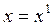,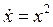we will have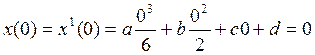, that’s why we eliminate one constant, it will be equal to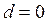.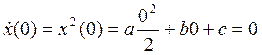, thus we eliminate the second constant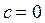.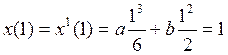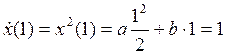From the system of two last equations we found the rest of constants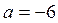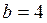5)  So, we have the control parameter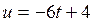and the equation of phase trajectory (fig. 3.1):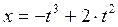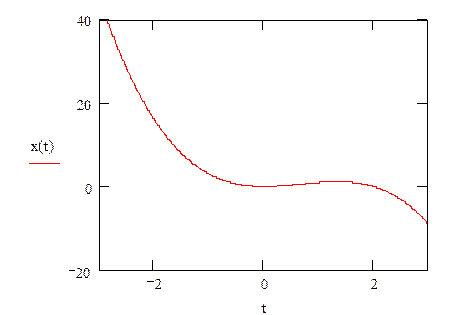Fig. 3.1 Phase trajectory

Тип:
Конспекты лекций
Размер файла:
108 Kb
Скачали:
0